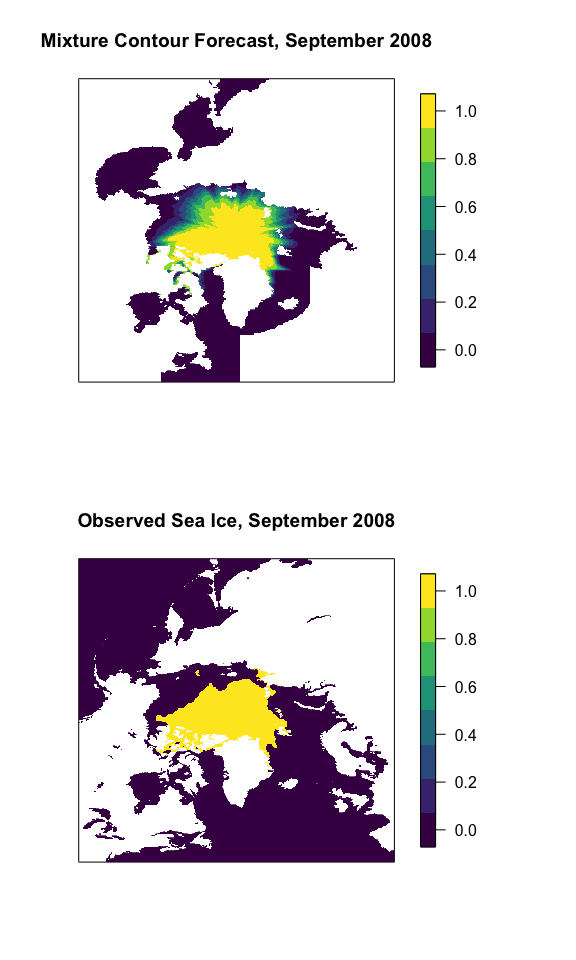Mixture Contour Forecasting

Introduction

This vignette introduces how to weight two probabilistic sea ice forecasts as in Probabilistic Forecasting of the Arctic Sea Ice Edge with Contour Modeling (Director et al. 2019+). In Director et al. (2019+), two forecasts are weighted to produce an overall forecast: one from the post-processed ensemble and the other from the climatology in the last ten years. We’ll fit the forecast for September 2008 using data from September 2005-2007 at a 2.5-month lead time.

Fitting Weights

The weights will be fit using observations and both forecast types during a 3-year training period. To get started, we’ll first load the IceCast R package.

library("IceCast")

The forecasts and observations should be arranged in an array of dimension of the number of training years x longitude x latitude. The post-processed ensemble forecast, the climatology forecast, and the observations for the training years for September 2005-2007 are stored in the package as clim_9_2005_2007, ppe_9_2005_2007, and obs_9_2005_2007. Their dimensions are all 3 x 304 x 448.

To find the weights, we input the model outputs and observations into the fit_weights function. We also need to supply a prop_area matrix, which is provided in the IceCast package for the Seas of the Arctic on a Polar Stereographic grid. This is just a matrix of dimension longitude by latitude that sums to 1. Each element gives the proportion of the total area contained in that grid box.

weight <- fit_weights(mod1 = clim_9_2005_2007, mod2 = ppe_9_2005_2007,
obs = obs_9_2005_2007, prop_area = prop_area)

Making a Forecast

To issue a MCF forecast for 2008, we use the weight computed in the previous step and matrices of dimension longitude x latitude that give the post-processed ensemble and climatology predictions. For September 2008, these values are stored in the package as ppe_9_2008 and clim_9_2008. We create the forecast as follows

MCF_9_2008 <- wght_mod(w = weight, mod1 = clim_9_2008, mod2 = ppe_9_2008)

Finally, we’ll plot the resulting forecast and the observation for comparison. To do this, we’ll also load a couple of packages for visualization:

library("fields")
library("viridis")
par(mfrow = c(2, 1), oma = c(0, 0, 0, 4))
image.plot(MCF_9_2008, main = "Mixture Contour Forecast, September 2008",
col = viridis(8), zlim = c(0, 1), xaxt = "n", yaxt = "n")
image.plot(obs_9_2008, main = "Observed Sea Ice, September 2008",
col = viridis(8), zlim = c(0, 1), xaxt = "n", yaxt = "n")